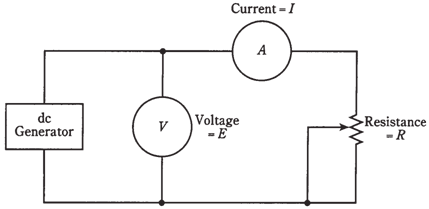## Resistance calculations Assignment Help

Assignment Help: >> Electrical Engineering - Resistance calculations

Resistance calculations

Ohms' Law is used to find out a resistance between 2 points in a direct current circuit, when the voltage and the current are known to us. For the following problems, imagine that the voltmeter and ammeter both scales in the Figure are visible, but the potentiometer is uncalibrated.Problem-1

If voltmeter reads 24 V and ammeter shows 3.0 A, what is the value of potentiometer?

Use formula E/I and plug in values directly, as they are expressed in volts and amperes: R = 24/3.0 = 8.0Ω.

Note that you can specify this value to 2 significant figures, the 8 and the zero, rather than saying simply 8Ω. This is because you are given both the voltage and the current to 2 significant figures. If ammeter reading had been given as 3A (meaning some value between 2 1/2 A and 3 1/2 A), you would only be entitled to express the answer as 8Ω. A zero can be a significant figure, as well as the digits 1 through 9.

Problem-2

What is value of resistance if the current is 18 mA and the voltage is 229 mV?

First, convert the values to amperes and volts. This gives I = 0.018 A and E = 0.229 V. Then plug into equation R=E/I= 0.229/0.018 = 13Ω. You are justified in giving your answer to 2 significant figures, as the current is only given to many digits.